# NCERT Solutions Class 5 Mathematics Chapter 3 How Many Squares

## Chapter 3 How Many Squares Class 5 Mathematics NCERT Solutions

Class 5 Mathematics students should refer to the following NCERT questions with answers for Chapter 3 How Many Squares in Class 5. These NCERT Solutions with answers for Class 5 Mathematics will come in exams and help you to score good marks

### Chapter 3 How Many Squares NCERT Solutions Class 5 Mathematics

1. Ziri tried to make some other tiles. She started with a square of 2 cm side and made shapes like these. Look at these carefully and find out:
Q. Which of these shapes will tile a floor (without any gaps)? Discuss. What is the area of these shapes?
Q. Make designs in your copy by tiling those shapes.
Q. Now you create your own new tiles out of a square. Can you do the same with a triangle? Try doing it.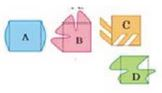Ans. The shapes C and D will tile a floor (without any gaps)
The area of each of these shapes (i.e. tiles) is
2 2 square cm = 4 square cm.

2. Make a pattern using your tile. Trace the shape to repeat it on a page, but remember there must be no gaps between them. Ziri made a pattern using her yellow tiles. (You know the area of her tile.)Q. How many tiles has she used?
Q. What is the area of the floor pattern Ziri has made here?
Ans. She has used 12 tiles.
The area of the floor pattern Ziri has made here is (12 3) square cm= 36 square cm.

3. Did you get all the 12 shapes using 5 squares?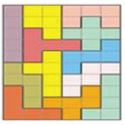Draw all the 12 shapes on a sheet of cardboard and cut them.
Ans. Yes, I get all the 12 shapes using 5 squares as shown above.
All the 12 shapes may be drawn on a sheet of cardboard and also cut them as desired.

4. Ziri went to a shop and was surprised to see the different designs of tiles on the floor. Aren’t these beautiful! Can you find the tile which is repeated to make each of these floor patterns? Circle a tile in each pattern.
Ans. A tile in each pattern is shown as encircle.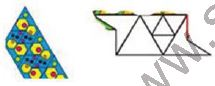5. After looking at the patterns Ziri wanted to make her own yellow tile. You too make a tile this way.
Step 1: Take a piece of cardboard or thick paper. Draw a square of side 3 cm on it.Step 2: Draw a triangle on any one of the sides of this square.Step 3: Draw another triangle of the same size on another side of the square.
But this time draw it inside the square.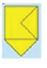Step 4: Cut this shape from the cardboard. Your tile is ready! What is it’s area?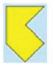Ans. It’s area is 3 3 square cm i.e. 9 square cm.

6. Here is a rectangle of area 20 square cm.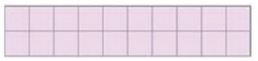a) Draw one straight line in this rectangle to divide it into two equal triangles.
What is the area of each of the triangles?
b) Draw one straight line in this rectangle to divide it into two equal rectangles. What is the area of each of the smaller rectangles?
c) Draw two straight lines in this rectangle to divide it into one rectangle and two equal triangles.
d) What is the area of the rectangle?
e) What is the area of each of the triangles?
Ans. (a) One straight line is drawn in the given rectangle to divide it in two equal triangle as: shown below.Area of each of the triangles is half of the given rectangle. Area of this rectangle is 20 square cm. So, the area of each of the triangles is 10 squares cm.

(b) One straight line is drawn in the given rectangle to divide it into two equal rectangles as shown below: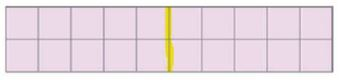Area of each of the smaller rectangles is half of the given rectangle. Area of the given rectangle is 20 square cm. So, the area of each of two rectangles is 10 square cm.
(c) Two straight lines are drawn in the given rectangle to divide it into one rectangle and two equal triangles as shown below: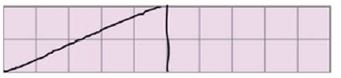(d) Area of the new rectangle is half of the given rectangle. Area of the given rectangle is 20 square cm. So, the area of the smaller rectangle is 10 square cm.
(e) Area of the each of the triangles is half of the rectangle of area 10 square cm.
So, the area of each of the triangles is 5 square cm.

 NCERT Solutions Class 5 Mathematics Chapter 1 The Fish Tale
 NCERT Solutions Class 5 Mathematics Chapter 2 Shapes and Angles
 NCERT Solutions Class 5 Mathematics Chapter 3 How Many Squares
 NCERT Solutions Class 5 Mathematics Chapter 4 Parts and Wholes
 NCERT Solutions Class 5 Mathematics Chapter 5 Does it look the same
 NCERT Solutions Class 5 Mathematics Chapter 6 Be my multiply
 NCERT Solutions Class 5 Mathematics Chapter 7 Can you see the pattern
 NCERT Solutions Class 5 Mathematics Chapter 8 Mapping Your Way
 NCERT Solutions Class 5 Mathematics Chapter 9 Boxes And Sketches
 NCERT Solutions Class 5 Mathematics Chapter 10 Tenths And Hundredths
 NCERT Solutions Class 5 Mathematics Chapter 11 Area and its Boundary
 NCERT Solutions Class 5 Mathematics Chapter 12 Smart Charts
 NCERT Solutions Class 5 Mathematics Chapter 13 Ways of Multiply and Divide
 NCERT Solutions Class 5 Mathematics Chapter 14 How Big How Heavy

## More Study Material

Tags

### NCERT Solutions Class 5 Mathematics Chapter 3 How Many Squares

NCERT Solutions Class 5 Mathematics Chapter 3 How Many Squares is available on our website www.studiestoday.com for free download in Pdf. You can read the solutions to all questions given in your Class 5 Mathematics textbook online or you can easily download them in pdf.

### Chapter 3 How Many Squares Class 5 Mathematics NCERT Solutions

The Class 5 Mathematics NCERT Solutions Chapter 3 How Many Squares are designed in a way that will help to improve the overall understanding of students. The answers to each question in Chapter 3 How Many Squares of Mathematics Class 5 has been designed based on the latest syllabus released for the current year. We have also provided detailed explanations for all difficult topics in Chapter 3 How Many Squares Class 5 chapter of Mathematics so that it can be easier for students to understand all answers.

#### NCERT Solutions Chapter 3 How Many Squares Class 5 Mathematics

Class 5 Mathematics NCERT Solutions Chapter 3 How Many Squares is a really good source using which the students can get more marks in exams. The same questions will be coming in your Class 5 Mathematics exam. Learn the Chapter 3 How Many Squares questions and answers daily to get a higher score. Chapter 3 How Many Squares of your Mathematics textbook has a lot of questions at the end of chapter to test the students understanding of the concepts taught in the chapter. Students have to solve the questions and refer to the step-by-step solutions provided by Mathematics teachers on studiestoday to get better problem-solving skills.

#### Chapter 3 How Many Squares Class 5 NCERT Solution Mathematics

These solutions of Chapter 3 How Many Squares NCERT Questions given in your textbook for Class 5 Mathematics have been designed to help students understand the difficult topics of Mathematics in an easy manner. These will also help to build a strong foundation in the Mathematics. There is a combination of theoretical and practical questions relating to all chapters in Mathematics to check the overall learning of the students of Class 5.

#### Class 5 NCERT Solution Mathematics Chapter 3 How Many Squares

NCERT Solutions Class 5 Mathematics Chapter 3 How Many Squares detailed answers are given with the objective of helping students compare their answers with the example. NCERT solutions for Class 5 Mathematics provide a strong foundation for every chapter. They ensure a smooth and easy knowledge of Revision notes for Class 5 Mathematics. As suggested by the HRD ministry, they will perform a major role in JEE. Students can easily download these solutions and use them to prepare for upcoming exams and also go through the Question Papers for Class 5 Mathematics to clarify all doubts

Where can I download latest NCERT Solutions for Class 5 Mathematics Chapter 3 How Many Squares

You can download the NCERT Solutions for Class 5 Mathematics Chapter 3 How Many Squares for latest session from StudiesToday.com

Can I download the NCERT Solutions of Class 5 Mathematics Chapter 3 How Many Squares in Pdf

Yes, you can click on the link above and download NCERT Solutions in PDFs for Class 5 for Mathematics Chapter 3 How Many Squares

Are the Class 5 Mathematics Chapter 3 How Many Squares NCERT Solutions available for the latest session

Yes, the NCERT Solutions issued for Class 5 Mathematics Chapter 3 How Many Squares have been made available here for latest academic session

How can I download the Chapter 3 How Many Squares Class 5 Mathematics NCERT Solutions

You can easily access the links above and download the Chapter 3 How Many Squares Class 5 NCERT Solutions Mathematics for each chapter

Is there any charge for the NCERT Solutions for Class 5 Mathematics Chapter 3 How Many Squares

There is no charge for the NCERT Solutions for Class 5 Mathematics Chapter 3 How Many Squares you can download everything free

How can I improve my scores by reading NCERT Solutions in Class 5 Mathematics Chapter 3 How Many Squares

Regular revision of NCERT Solutions given on studiestoday for Class 5 subject Mathematics Chapter 3 How Many Squares can help you to score better marks in exams

Are there any websites that offer free NCERT solutions for Chapter 3 How Many Squares Class 5 Mathematics

Yes, studiestoday.com provides all latest NCERT Chapter 3 How Many Squares Class 5 Mathematics solutions based on the latest books for the current academic session

Can NCERT solutions for Class 5 Mathematics Chapter 3 How Many Squares be accessed on mobile devices

Yes, studiestoday provides NCERT solutions for Chapter 3 How Many Squares Class 5 Mathematics in mobile-friendly format and can be accessed on smartphones and tablets.

Are NCERT solutions for Class 5 Chapter 3 How Many Squares Mathematics available in multiple languages

Yes, NCERT solutions for Class 5 Chapter 3 How Many Squares Mathematics are available in multiple languages, including English, Hindi